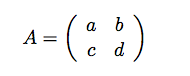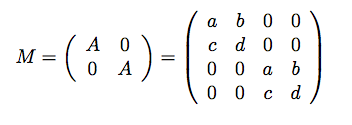# How to combine TMatrixD?

Hello!

Just wondering if there’s an easy way to combine matrices in ROOT?

For example, if I have given number of matrices similar to this one (but not always 2x2):and want to combine them to give a new matrix, MIs there a function that will easily do this?

Thanks!
Sam

Hi Sam,

Suppose you want to combine matrix x1 and x2 as blocks
as you indicated in your example:

``````const Int_t nrow1 = x1.GetNrows();
const Int_t nrow2 = x2.GetNrows();
const Int_t ncol1 = x1.GetNcols();
const Int_t ncol2 = x2.GetNcols();

const Int_t nrow3 = nrow1+nrow2;
const Int_t ncol3 = ncol1+ncol2;

TMatrixD x3(nrow3,ncol3);
TMatrixDSub(x3,       0,nrow1-1,     0,ncol1-1) = x1;
TMatrixDSub(x3,nrow1,nrow3-1,ncol1,ncol3-1) = x2;``````

Thanks, that’s really helpful. My “combine” function needs to be able to handle any number of matrices, so I guess I’ll have to have a think about that; this is a really good starting point though! ThanksIf anyone is interested, I’ve written a function to combine matrices:

``````
TMatrixDSym PrepInputs::CombineMatrices(std::vector<TMatrixDSym> input_matrices){

// Get the size of the combined matrix:
int total_rows = 0;
for(int m = 0; m < (int) input_matrices.size(); m++){
total_rows += input_matrices[m].GetNrows();
}

// Combine the input matrices together
TMatrixDSym combined_matrix(total_rows);
int sum_nrows = 0;
for(int m = 0; m < (int) input_matrices.size(); m++){
int nrows = input_matrices[m].GetNrows();
int lower_bound = sum_nrows;
int upper_bound = sum_nrows + nrows - 1;
TMatrixDSub(combined_matrix, lower_bound, upper_bound, lower_bound, upper_bound) = input_matrices[m];
sum_nrows += nrows;
}

return combined_matrix;
}

``````